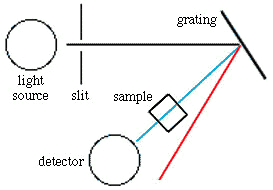Beer-Lambert Law and Visible Light Spectrometers

## Beer-Lambert Law and Visible Light Spectrometers

Spectroscopic Measurements and the Beer-Lambert Law

When a beam of radiation (light) passes through a substance or a solution, some of the light may be absorbed and the remainder transmitted through the sample. The ratio of the intensity of the light entering the sample (Io) to that exiting the sample (It) at a particular wavelength is defined as the transmittance (T). This is often expressed as the percent transmittance (%T), which is simply the transmittance multiplied by 100. The absorbance (A) of a sample is the negative logarithm of the transmittance.

% T = (Io / It ) x 100

A = - log (T)

The absorbance of a sample at a given wavelength is proportional to the absorptivity of the substance (a constant at each wavelength), the path length (the distance the light travels through the sample) and, in many instances, the concentration of the absorbing substance. In these cases the Beer-Lambert Law holds:

 A = a * b * c where a = the absorptivity of the substance b = path length c = concentration of the substance

Commonly, a and b are constant for a given set of experiments so that a plot of the sample absorbance vs. the concentration of the absorbing substance is a straight line. In practice, a calibration curve is prepared by plotting the absorbance of a series of standard samples as a function of their concentration. If the absorbance of an unknown sample is then measured, the concentration of the absorbing component can be determined from this graph.

See an example of how to use the Beer-Lambert Law.

UV-Visible spectrometers

UV-Visible spectrometers are composed of four basic parts: the light source, a monochromator, the sample holder and detector. The most commonly used light sources produce a continuous spectrum of radiation. As the Beer-Lambert Law does not hold for multiple wavelengths, a monochromator (historically this contained a prism, but currently gratings are used) is used to select a single wavelength of light. The light leaving the monochromator is directed through the sample cell which contains the solution to be analyzed. A detector (normally a photo- or photomultiplier tube) is used to determine the intensity of the radiation passing through the sample.A schematic of a visible light spectrometer.
The wavelength of light is selected by rotating the grating.

Continue on to how to make absorbance measurements using a Spectronic-20 spectrometer.